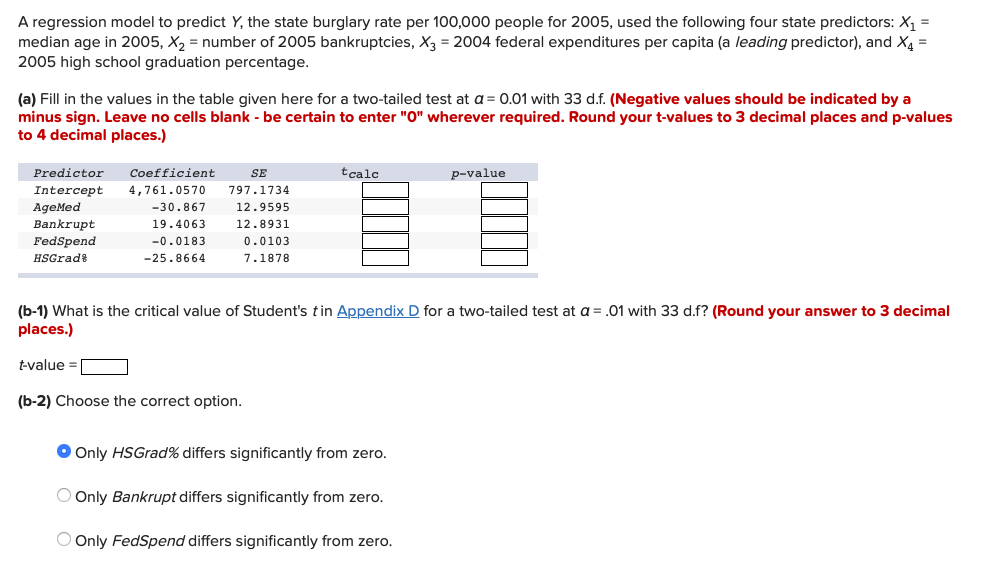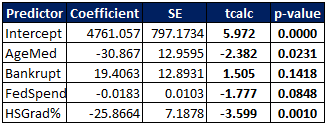# A regression model to predict Y, the state burglary rate per 100,000 people for 2005, used...A regression model to predict Y, the state burglary rate per 100,000 people for 2005, used the following four state predictors: X1 = median age in 2005, X2 = number of 2005 bankruptcies, X3 = 2004 federal expenditures per capita (a leading predictor), and X4 = 2005 high school graduation percentage. (a) Fill in the values in the table given here for a two-tailed test at a = 0.01 with 33 d.f. (Negative values should be indicated by a minus sign. Leave no cells blank - be certain to enter "0" wherever required. Round your t-values to 3 decimal places and p-values to 4 decimal places.) calc p-value Predictor Intercept AgeMed Bankrupt FedSpend HSGrad: Grad Coefficient 4,761.0570 -30.867 19.4063 -0.0183 -25.8664 SE 797.1734 12.9595 12.8931 0.0103 7.1878 (6-1) What is the critical value of Student's tin Appendix D for a two-tailed test at a = .01 with 33 d.f? (Round your answer to 3 decimal places.) t-value =D (6-2) Choose the correct option. Only HSGrad% differs significantly from zero. Only Bankrupt differs significantly from zero. Only FedSpend differs significantly from zero.

a) t values will be the ratio of Coefficient and SE and then, p - value will be two tailed value corresponding to that t at 33 d.f. Hence,

The complete table will be:b) t value = 2.733

c) Option A is correct.

#### Earn Coin

Coins can be redeemed for fabulous gifts.